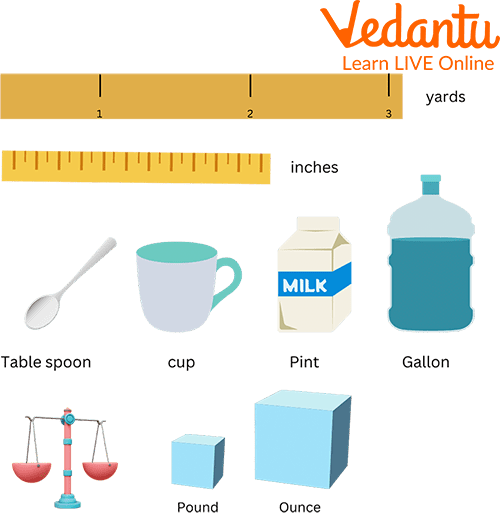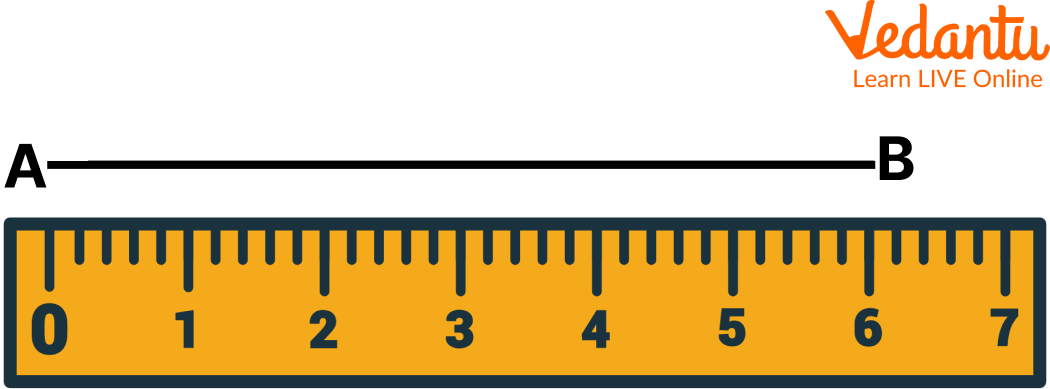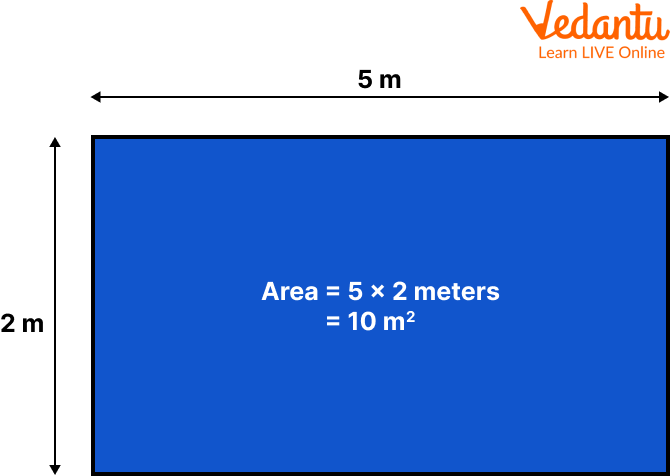Courses
Courses for Kids
Free study material
Free LIVE classes
More

# Length, Area and CapacityLIVE
Join Vedantu’s FREE Mastercalss

## Introduction to the Definition of Measurement in Math

Measurement is finding the number which shows the size or amount of something. It can also be described as a technique through which the properties of an object are determined to a standard quantity. It is also referred to as the process or result of determining the magnitude of quantity such as length or mass, relative to a unit of measurement, such as meter or kilogram.Varieties of Measuring Units.

The units of measurement are the collection of standard and other units that are used to measure various physical quantities. It is a tool to measure and compare different things. Comparison becomes easy when all the units for the measurement are the same.

## Definition of Unit in Maths

Units are the tools to measure and compare different things. Comparison becomes easy when all the units for the measurement are the same. Different units can be classified depending on their use.

A Few of the Units Can be Classified As:

Length: The length evaluates the space or distance between two points or it is the amount of space between two points. For example the distance between the school and house, or the distance from one end of the road to another end.

Below a line having a length AB of 6cm, measured by a ruler is the examples of measurement.Ruler

The principal unit for measuring length can be described as the following:

 10 millimeters (mm) 1 centimeter (cm) 10 centimeters (cm) 100 millimeters (mm) 100 centimeters (cm) 1 meter (m) 1000 meters (m) 1 kilometer (km) 1 feet (ft.) 12 Inch 1 Mile 5280 Foot

Area: Area is the term which is used to describe the amount of space taken up by a 2D shape or surface. The area is measured in square units. The area is calculated by multiplying the length of a shape by its width.Example of Area

Capacity: Capacity is the maximum amount that something can contain, and volume is the amount of space that a substance or object occupies. The term capacity is used to measure how much fluid fits inside the container. It is measured in milliliters (ml), liters (l), or in imperial, pints and gallons.

1 Liter = 1000 milliliters

1 Kiloliter = 1000 litersDifferent Objects Showing the Capacity are the Examples of Measurement

## Scales of Measurement

1. Nominal Scale of Measurement: It defines the identity property of data. It contains various characteristics but does not carry any form of numerical meaning. The data can be placed into categories but it cannot be added, subtracted, divided, or multiplied from one another.

2. Ordinal Scale of Measurement: The ordinal scale defines data that is placed in a specific order. These values cannot be added to or subtracted from.

3. Interval Scale of Measurement: It contains properties of nominal and ordered data, but the difference between data points can be quantified. This type of data shows both the order of the variables and the exact differences between the variables. They can be added to or subtracted from each other, but not multiplied or divided.

4. Ratio Scale of Measurement: This includes properties from all the scales of measurement. The data is nominal and defined by an identity, which can be classified in order, contains intervals, and can be broken down into the exact value. Examples of ratio scales are weight, height, and distance. It can be added, subtracted, divided, and multiplied.

## Properties of Measurement

• Identity: It refers to each value that has a unique meaning.

• Magnitude: It determines whether the object is larger or smaller than other objects of the same kind.

• Equal Intervals: It means that the differences between numbers (units) anywhere on the scale are the same.

• A minimum value of zero: It means that the scale has a true zero point.

## Solved Examples

Q1. Convert 5km into meters.

Ans: 1km = 1000m

5km = (5 x 1000)m

= 5000m

So 5km in meteres is 5000m.

Q2. Convert 730mm into centimeters.

Ans: 1cm = 10mm

730mm = (730 \$\div\$ 10)cm

= 73cm

Therefore, 730mm into cm is 73.

Q3. How many feet are there in 60 inches?

Ans: 1 feet = 12inches

60inches = (60 \$\div\$12)inches

= 5 feet

So 60 inches in feet is 5 feet.

## Practice Questions

Q 1. Convert:

1. 35 meters = _____cm

2. 20 litres = _____ml

3. 12 feet = _____ inches

Ans: 1. 3500 cm

2. 20000 ml

3. 144 inches

Q 2. Add 350kg 20g and 25kg 45 grams.

Ans: 375kg and 65g

Q3. Subtract 200g from 1 kg.

Ans: 800g

## Summary

In this article, we learned that measurements play an important role in enhancing the mathematical skills of the students as it is a very basic topic, which is very important to remember as it helps in higher classes. Length, area, and capacity are very basic topics that help in measuring the object very accurately and precisely making it a lot of fun while learning. Depending upon the size of the object it can be measured through different prefixes like liters, kilograms, centimeters, etc.

Last updated date: 29th Sep 2023
Total views: 80.7k
Views today: 1.80k

## FAQs on Length, Area and Capacity

1. What are the instruments through which length can be measured?

There are various instruments through which length is measured and they are yardsticks, feet scales, rulers, measuring tape, meter scales, etc.

2. How to find the area of irregular shapes?

The area of irregular shapes can be found by dividing the shape into unit squares. When the shape does not occupy the complete unit square, we can approximate and find its value.

3. What is DG in Maths?

In maths DG refers to the decigram which is one of the units of measurement of weight. It is used to measure small quantities.

1 gram = 10 decigram